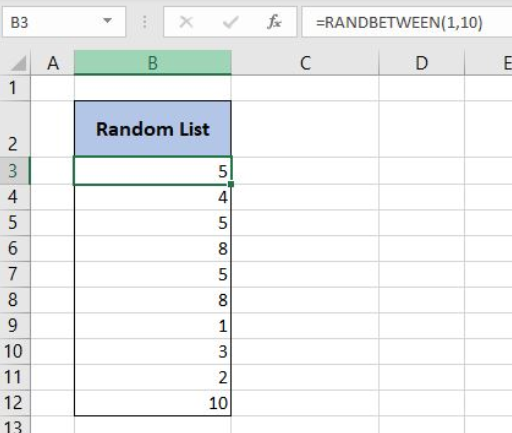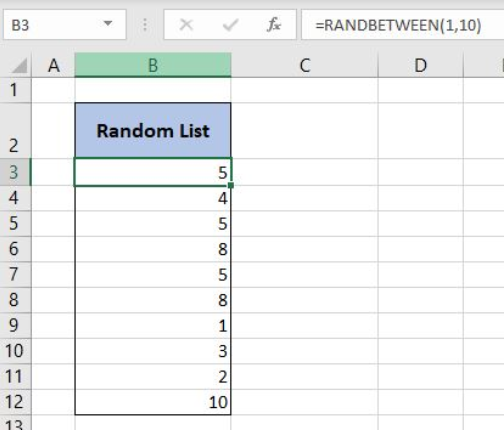Get instant live expert help with Excel or Google Sheets“My Excelchat expert helped me in less than 20 minutes, saving me what would have been 5 hours of work!”

#### Post your problem and you’ll get expert help in seconds.

Your message must be at least 40 characters
Our professional experts are available now. Your privacy is guaranteed.

# Create and Use a Random List Generator in Excel

Excel allows us to create a random list generator using the RANDBETWEEN function. This step by step tutorial will assist all levels of Excel users to create and use a random list generator in Excel.Figure 1. The final result of the formula

## Syntax of the RANDBETWEEN Formula

The generic formula for the RANDBETWEEN function is:

`=RANDBETWEEN(bottom, top)`

The parameters of the RANDBETWEEN function are:

• bottom – a value from which we want to get a random value
• top – a value to which we want to get a random value.

## Create a Random List Generator Using the RANDBETWEEN Formula

In the example, we want to generate a list of values between 1 and 10.

The formula for RANDBETWEEN in B3 looks like:

`=RANDBETWEEN(1, 10)`

The parameter bottom of the RANDBETWEEN function is 1 and the top is 10, as we want to get a list of values 1-10.

To apply the formula, we need to follow these steps:

• Select cell B3 and click on it
• Insert the formula: `=RANDBETWEEN(1, 10)`
• Press enter
• Drag the formula down to the other cells in the column by clicking and dragging the little “+” icon at the bottom-right of the cell.Figure 2. Using the RANDBETWEEN formula

After creating this formula, we can see that in column B we have the list of numbers 1-10.

Most of the time, the problem you will need to solve will be more complex than a simple application of a formula or function. If you want to save hours of research and frustration, try our live Excelchat service! Our Excel Experts are available 24/7 to answer any Excel question you may have. We guarantee a connection within 30 seconds and a customized solution within 20 minutes.

Solution examplesI'm Apply a =RANDBETWEEN formula in one cells want to apply Formula to entire column and run formula when i hit the entire on the particular cell
Solved by M. S. in 20 minsHelp! In a coin toss I have a 1 in 2 (fifty-fifty) chance of getting heads or tails. I need a formula to determine my chance of getting 4 heads in a row or 4 tails in a row out of 10 coin tosses. If I get 4 in a row I win. What formula can I use to determine my chances of winning if I repeat the 10 tosses for hundreds or thousands of times? I get as far as =RANDBETWEEN(0,1) but I have not been able to figure out what formula to use to determine if there are 4 in a row in a group of 10 tosses, times thousands of groups with 10 tosses in each group. Thank-you for any help you can provide.
Solved by V. F. in 51 minsI have 4 column. when i fill the first 3 column. in 4th column i need a unique id number and i generate unique id number using RANDBETWEEN formula [ =CHAR(RANDBETWEEN(65,90))&CHAR(RANDBETWEEN(65,90))&RANDBETWEEN(1000,9900) ] but i want ..... when i'm filling the 3 column after that 4th column run this formula and generate unique id automatically
Solved by X. Q. in 20 minswhen i fill column A and B then C column generate Unique ID using RandBetween Formula {=CHAR(RANDBETWEEN(65,90))&CHAR(RANDBETWEEN(65,90))&RANDBETWEEN(1000,9900)} ...
Solved by S. Q. in 20 mins